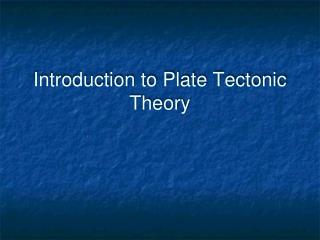DownloadDownload PresentationIntroduction to Plate Tectonic Theory

# Introduction to Plate Tectonic Theory

Télécharger la présentation## Introduction to Plate Tectonic Theory

- - - - - - - - - - - - - - - - - - - - - - - - - - - E N D - - - - - - - - - - - - - - - - - - - - - - - - - - -
##### Presentation Transcript

1. Introduction to Plate Tectonic Theory

2. Introduction • Plate tectonics is the theory that Earth's outer layer is made up of plates, which have moved throughout Earth's history. • The theory explains the how and why behind mountains, volcanoes, and earthquakes.

3. Pangaea • 225 million years ago, all the major continents formed one giant supercontinent, called Pangaea. • Initiated by heat building up underneath the continent, Pangaea began to split apart, around 200 million years ago. These continents are still on the move today.

4. Theories of Plate Motion • Exactly what drives plate tectonics is not known. • One theory is that convection within the Earth's mantle pushes the plates. • Another theory is that gravity is pulling the older, heavier ocean floor with more force than the newer, lighter seafloor.

5. Plate Motion • Plate tectonic activity takes place at four types of boundaries: • Divergent boundaries • Convergent boundaries • Collisional boundaries • Transform boundaries

6. Divergent Boundaries • Also known as spreading boundary, it occurs where two plates move apart, allowing magma, to rise from the Earth's interior to fill in the gap. • The two plates move away from each other like two conveyor belts moving in opposite directions.

7. Convergent Boundaries • Also known as subduction boundary, it occurs where one plate slides under another as the two are pushed together. • If there is land at the edge of one of these plates, the ocean plate will subduct, or slide under that plate.

8. Collisional Boundaries • A collisional boundary occurs where two land masses on plates are pushed together. • Trying to occupy the same space, the land masses buckle and fold, creating mountain ranges.

9. Transform Boundaries • A transform boundary occurs where two plates slide against each other. • Rather than sliding smoothly, the plates build up tension, then release the tension with a spurt of movement called an earthquake

10. Birth of a Theory video questions • What led Wegener to his notion of continental drift? • What is and where does sea floor spreading occur? • How did paleomagnetism help support the notion of sea floor spreading? • What is a transform fault? • Why is plate tectonic theory called a “model that describes everything”?